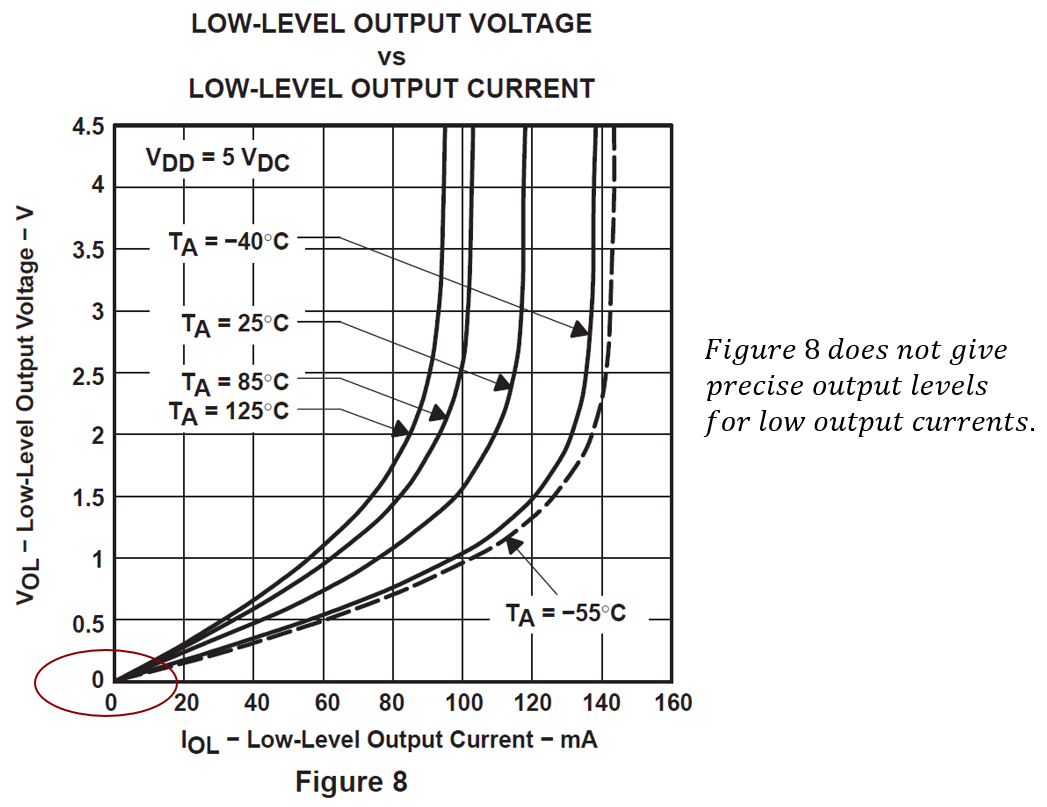If you have a related question, please click the "Ask a related question" button in the top right corner. The newly created question will be automatically linked to this question.

TLV2462A-Q1: Low Level Output Voltage @ Low Output Current

Part Number: TLV2462A-Q1

Hi there,

ive got a question regarding the low level output voltage of this opamp (TLV2462A-Q1).

in the datasheet the low level output voltage over output current is written for higher current than in my application.

so the precise question is: how high is the low level output voltage @ 500uA• Hi Kevin,

as the curves start from the zero point (origin) of the diagram, a linear interpolation can help to find an estimation of the low level output voltage at 500µA:

0.5V / 30mA = x / 500µA

->  x = 8.3mV.

Also, as the diagram only shows the typical behaviour, it's wise to take into account some manufacturing tolerances.

You can verify this result by a simple measurement. And by checking a handful of chips you can get an estimate about the statistical spreading of low level output voltage due to manufacturing tolerences.

Kai

• Kevin,

Kai covered typical from chart. I'll cover worst case from EC table in section 6.10 of data sheet.

Over full range of temperature, VOL is 0.2V and IOL of 2.5mA (worst case will be 125C)

VOL / IOL = ROUT = 80 ohms.  0.5mA * 80 ohms is 40 mV